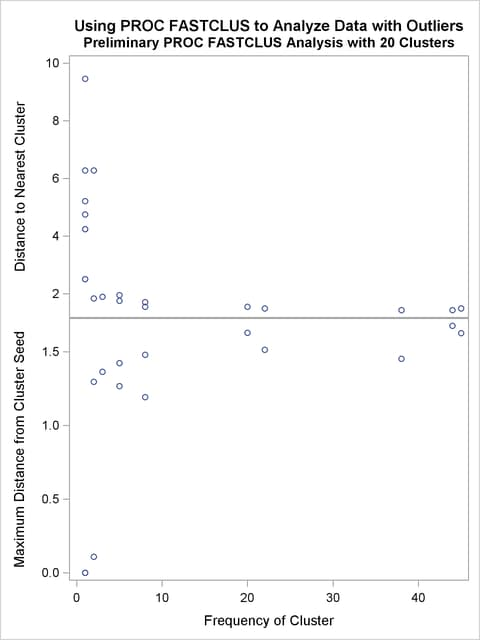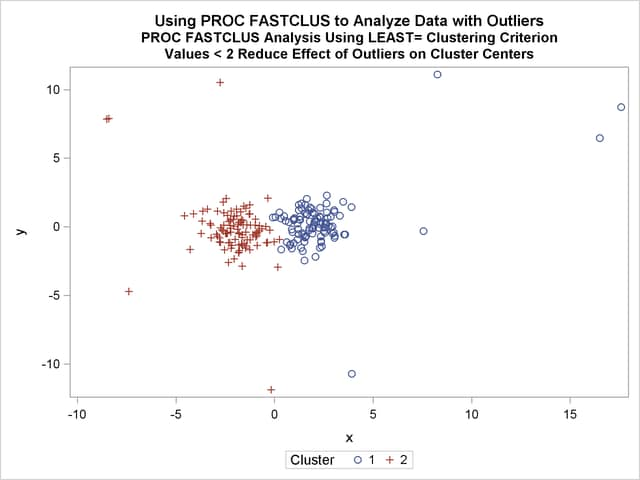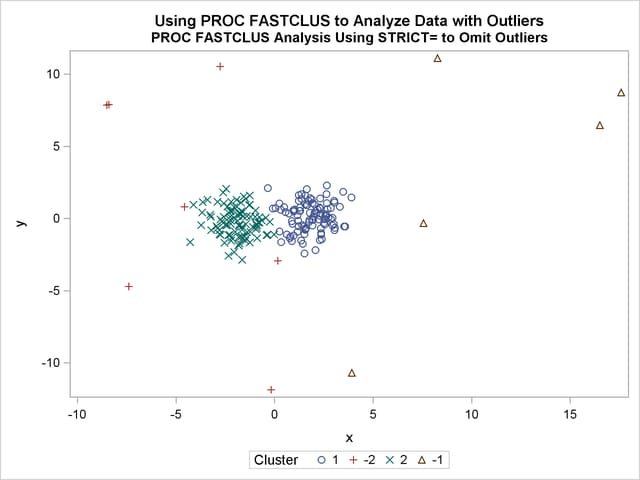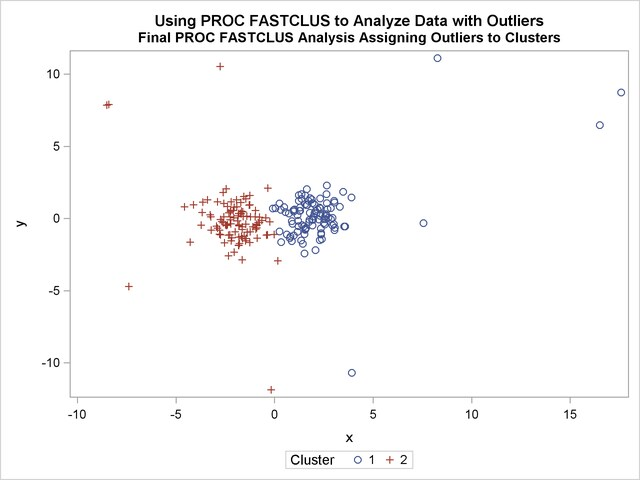Previous Page | Next Page

 The FASTCLUS Procedure

Example 34.2 Outliers

This example involves data artificially generated to contain two clusters and several severe outliers. A preliminary analysis specifies 20 clusters and outputs an OUTSEED= data set to be used for a diagnostic plot. The exact number of initial clusters is not important; similar results could be obtained with 10 or 50 initial clusters. Examination of the plot suggests that clusters with more than five (again, the exact number is not important) observations can yield good seeds for the main analysis. A DATA step deletes clusters with five or fewer observations, and the remaining cluster means provide seeds for the next PROC FASTCLUS analysis.

Two clusters are requested; the LEAST= option specifies the mean absolute deviation criterion (LEAST=1). Values of the LEAST= option less than 2 reduce the effect of outliers on cluster centers.

The next analysis also requests two clusters; the STRICT= option is specified to prevent outliers from distorting the results. The STRICT= value is chosen to be close to the _GAP_ and _RADIUS_ values of the larger clusters in the diagnostic plot; the exact value is not critical.

A final PROC FASTCLUS run assigns the outliers to clusters.

The following SAS statements implement these steps, and the results are displayed in Output 34.2.3 through Output 34.2.8. First, an artificial data set is created with two clusters and some outliers. Then PROC FASTCLUS is run with many clusters to produce an OUTSEED= data set. A diagnostic plot using the variables _GAP_ and _RADIUS_ is then produced using the SGSCATTER procedure. The results from these steps are shown in Output 34.2.1 and Output 34.2.2.

data x;
title 'Using PROC FASTCLUS to Analyze Data with Outliers';
drop n;
do n=1 to 100;
x=rannor(12345)+2;
y=rannor(12345);
output;
end;
do n=1 to 100;
x=rannor(12345)-2;
y=rannor(12345);
output;
end;
do n=1 to 10;
x=10*rannor(12345);
y=10*rannor(12345);
output;
end;
run;

title2 'Preliminary PROC FASTCLUS Analysis with 20 Clusters';
proc fastclus data=x outseed=mean1 maxc=20 maxiter=0 summary;
var x y;
run;

proc sgscatter data=mean1 ;
compare y=(_gap_ _radius_) x=_freq_ ;
run;

Output 34.2.1 Preliminary Analysis of Data with Outliers Using PROC FASTCLUS
 Using PROC FASTCLUS to Analyze Data with Outliers Preliminary PROC FASTCLUS Analysis with 20 Clusters

The FASTCLUS Procedure
Replace=FULL Radius=0 Maxclusters=20 Maxiter=0

 Criterion Based on Final Seeds = 0.6873

Cluster Summary
Cluster Frequency RMS Std Deviation Maximum Distance
from Seed
to Observation
Exceeded
Nearest Cluster Distance Between
Cluster Centroids
1 8 0.4753 1.1924   19 1.7205
2 1 . 0   6 6.2847
3 44 0.6252 1.6774   5 1.4386
4 1 . 0   20 5.2130
5 38 0.5603 1.4528   3 1.4386
6 2 0.0542 0.1085   2 6.2847
7 1 . 0   14 2.5094
8 2 0.6480 1.2961   1 1.8450
9 1 . 0   7 9.4534
10 1 . 0   18 4.2514
11 1 . 0   16 4.7582
12 20 0.5911 1.6291   16 1.5601
13 5 0.6682 1.4244   3 1.9553
14 1 . 0   7 2.5094
15 5 0.4074 1.2678   3 1.7609
16 22 0.4168 1.5139   19 1.4936
17 8 0.4031 1.4794   5 1.5564
18 1 . 0   10 4.2514
19 45 0.6475 1.6285   16 1.4936
20 3 0.5719 1.3642   15 1.8999

 Pseudo F Statistic = 207.58

 Observed Over-All R-Squared = 0.95404

 Approximate Expected Over-All R-Squared = 0.96103

 Cubic Clustering Criterion = -2.503

WARNING: The two values above are invalid for correlated variables.

Output 34.2.2 Preliminary Analysis of Data with Outliers: Plot Using and PROC SGSCATGTERIn the following SAS statements, a DATA step is used to remove low frequency clusters, then the FASTCLUS procedure is run again, selecting seeds from the high frequency clusters in the previous analysis using LEAST=1 clustering criterion. The results are shown in Output 34.2.3 and Output 34.2.4.

data seed;
set mean1;
if _freq_>5;
run;

title2 'PROC FASTCLUS Analysis Using LEAST= Clustering Criterion';
title3 'Values < 2 Reduce Effect of Outliers on Cluster Centers';
proc fastclus data=x seed=seed maxc=2 least=1 out=out;
var x y;
run;

proc sgplot data=out;
scatter y=y x=x /group=cluster;
run;

Output 34.2.3 Analysis of Data with Outliers Using the LEAST= Option
 Using PROC FASTCLUS to Analyze Data with Outliers PROC FASTCLUS Analysis Using LEAST= Clustering Criterion Values < 2 Reduce Effect of Outliers on Cluster Centers

The FASTCLUS Procedure
Replace=FULL Radius=0 Maxclusters=2 Maxiter=20 Converge=0.0001 Least=1

Initial Seeds
Cluster x y
1 2.794174248 -0.065970836
2 -2.027300384 -2.051208579

 Minimum Distance Between Initial Seeds = 6.80671

 Preliminary L(1) Scale Estimate = 2.79658

 Number of Bins = 100

Iteration History
Iteration Criterion Maximum Bin Size Relative Change in Cluster
Seeds
1 2
1 1.3983 0.2263 0.4091 0.6696
2 1.0776 0.0226 0.00511 0.0452
3 1.0771 0.00226 0.00229 0.00234
4 1.0771 0.000396 0.000253 0.000144
5 1.0771 0.000396 0 0

 Convergence criterion is satisfied.

 Criterion Based on Final Seeds = 1.0771

Cluster Summary
Cluster Frequency Mean Absolute
Deviation
Maximum Distance
from Seed
to Observation
Exceeded
Nearest Cluster Distance Between
Cluster Medians
1 102 1.1278 24.1622   2 4.2585
2 108 1.0494 14.8292   1 4.2585

Cluster Medians
Cluster x y
1 1.923023887 0.222482918
2 -1.826721743 -0.286253041

Mean Absolute Deviations from Final
Seeds
Cluster x y
1 1.113465261 1.142120480
2 0.890331835 1.208370913

Output 34.2.4 Analysis Plot of Data with OutliersThe FASTCLUS procedure is run again, selecting seeds from high frequency clusters in the previous analysis. STRICT= prevents outliers from distorting the results. The results are shown in Output 34.2.5 and Output 34.2.6.

title2 'PROC FASTCLUS Analysis Using STRICT= to Omit Outliers';
proc fastclus data=x seed=seed
maxc=2 strict=3.0 out=out outseed=mean2;
var x y;
run;

proc sgplot data=out;
scatter y=y  x=x /group=cluster ;
run;

Output 34.2.5 Cluster Analysis with Outliers Omitted: PROC FASTCLUS SGPLOT
 Using PROC FASTCLUS to Analyze Data with Outliers PROC FASTCLUS Analysis Using STRICT= to Omit Outliers

The FASTCLUS Procedure
Replace=FULL Radius=0 Strict=3 Maxclusters=2 Maxiter=1

Initial Seeds
Cluster x y
1 2.794174248 -0.065970836
2 -2.027300384 -2.051208579

 Criterion Based on Final Seeds = 0.9515

Cluster Summary
Cluster Frequency RMS Std Deviation Maximum Distance
from Seed
to Observation
Exceeded
Nearest Cluster Distance Between
Cluster Centroids
1 99 0.9501 2.9589   2 3.7666
2 99 0.9290 2.8011   1 3.7666

12 Observation(s) were not assigned to a cluster  because the minimum distance to a cluster seed  exceeded the STRICT= value.

Statistics for Variables
Variable Total STD Within STD R-Square RSQ/(1-RSQ)
x 2.06854 0.87098 0.823609 4.669219
y 1.02113 1.00352 0.039093 0.040683
OVER-ALL 1.63119 0.93959 0.669891 2.029303

 Pseudo F Statistic = 397.74

 Approximate Expected Over-All R-Squared = 0.60615

 Cubic Clustering Criterion = 3.197

WARNING: The two values above are invalid for correlated variables.

Cluster Means
Cluster x y
1 1.825111432 0.141211701
2 -1.919910712 -0.261558725

Cluster Standard Deviations
Cluster x y
1 0.889549271 1.006965219
2 0.852000588 1.000062579

Output 34.2.6 Cluster Analysis with Outliers Omitted: Plot Using PROC SGPLOTFinally, the FASTCLUS procedure is run one more time with zero iterations to assign outliers and tails to clusters. The results are show in Output 34.2.7 and Output 34.2.8.

title2 'Final PROC FASTCLUS Analysis Assigning Outliers to Clusters';
proc fastclus data=x seed=mean2 maxc=2 maxiter=0 out=out;
var x y;
run;

proc sgplot data=out;
scatter y=y x=x /group=cluster ;
run;

Output 34.2.7 Cluster Analysis with Outliers Omitted: PROC FASTCLUS
 Using PROC FASTCLUS to Analyze Data with Outliers Final PROC FASTCLUS Analysis Assigning Outliers to Clusters

The FASTCLUS Procedure
Replace=FULL Radius=0 Maxclusters=2 Maxiter=0

Initial Seeds
Cluster x y
1 1.825111432 0.141211701
2 -1.919910712 -0.261558725

 Criterion Based on Final Seeds = 2.0594

Cluster Summary
Cluster Frequency RMS Std Deviation Maximum Distance
from Seed
to Observation
Exceeded
Nearest Cluster Distance Between
Cluster Centroids
1 103 2.2569 17.9426   2 4.3753
2 107 1.8371 11.7362   1 4.3753

Statistics for Variables
Variable Total STD Within STD R-Square RSQ/(1-RSQ)
x 2.92721 1.95529 0.555950 1.252000
y 2.15248 2.14754 0.009347 0.009435
OVER-ALL 2.56922 2.05367 0.364119 0.572621

 Pseudo F Statistic = 119.11

 Approximate Expected Over-All R-Squared = 0.4909

 Cubic Clustering Criterion = -5.338

WARNING: The two values above are invalid for correlated variables.

Cluster Means
Cluster x y
1 2.280017469 0.263940765
2 -2.075547895 -0.151348765

Cluster Standard Deviations
Cluster x y
1 2.412264861 2.089922815
2 1.379355878 2.201567557

Output 34.2.8 Cluster Analysis with Outliers Omitted: Plot Using PROC SGPLOTPrevious Page | Next Page | Top of Page